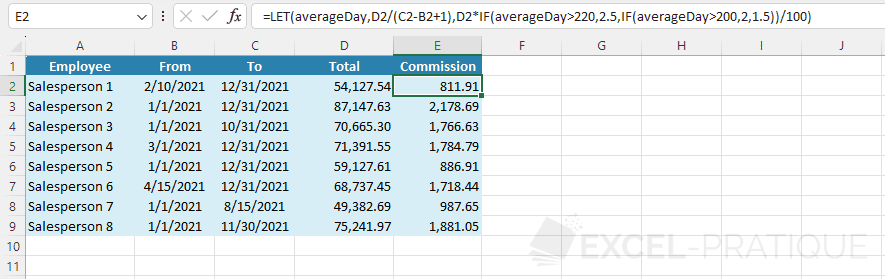# Excel Function: LET

The Excel function LET assigns a name to the results of calculations to store and reuse values within a formula.

The main purpose of this function is to avoid calculating the same result several times in the same formula. Instead, the LET function allows you to calculate this result once and assign a name to this result to reuse it in the formula.

Usage:

`=LET(name, value, formula)`

or

`=LET(name_1, value_1, name_2, value_2, ..., formula)`

## Simple usage example

In this example, the following formula multiplies the cell value by 4:

``=A2*2+A2*2``

There is of course much simpler way to multiply a number by 4, but the goal here is to have a simple formula with a repeated calculation.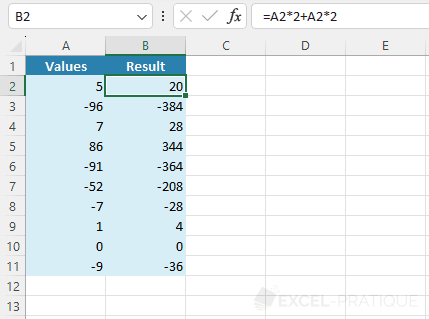To avoid the repetition of A2*2, store the result of this calculation using the LET function and assign it a name, for example x:

``=LET(x,A2*2,``

Then add the initial formula A2*2+A2*2 replacing A2*2 with x:

``=LET(x,A2*2,x+x)``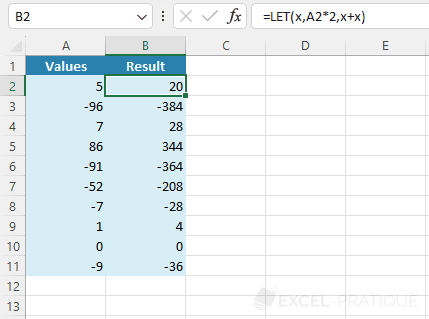If needed, you can store the results of several additional calculations in the same LET function, for example: =LET(a,A1,b,A2,c,A3,a+b+c)

## Usage example

In this new example, the goal is to calculate the commissions of salespeople by applying a rate based on the average turnover per day.

Column E contains here the formula which calculates the average per day:

``=D2/(C2-B2+1)``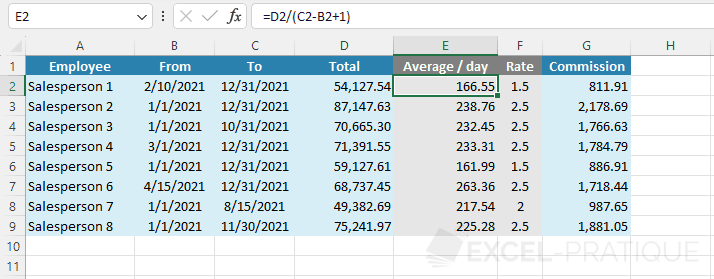The formula in column F determines the commission rate to apply using IF functions:

``=IF(E2>220,2.5,IF(E2>200,2,1.5))``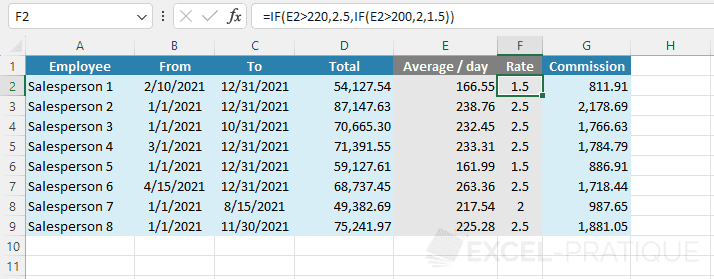And the commission is calculated in column G using the following formula:

``=D2*F2/100``

To directly display the amount of commissions, without going through intermediate columns (the gray columns), it is possible to combine these formulas into a single formula:

``=D2*IF(D2/(C2-B2+1)>220,2.5,IF(D2/(C2-B2+1)>200,2,1.5))/100``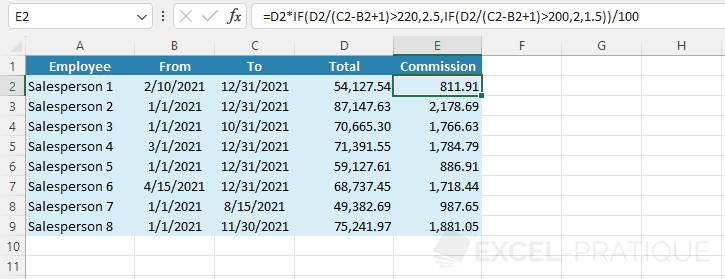To avoid calculating the average per day D2/(C2-B2+1) twice and make the formula a bit more readable, store the result of the average calculation using the LET function:

``=LET(averageDay,D2/(C2-B2+1),``

And add the complete formula as the third argument replacing D2/(C2-B2+1) with the defined name:

``=LET(averageDay,D2/(C2-B2+1),D2*IF(averageDay>220,2.5,IF(averageDay>200,2,1.5))/100)``